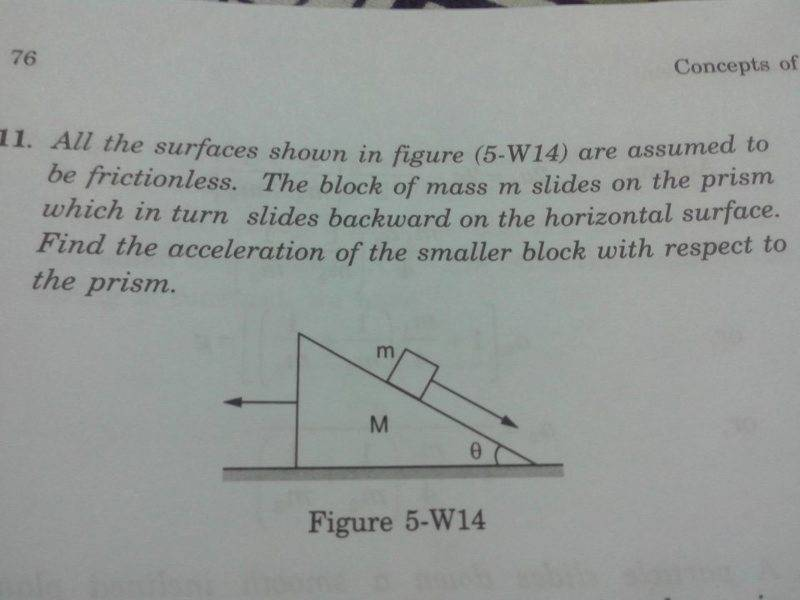# Newton's laws -- Block sliding on a Prism

• Prathamesh
In summary, the block slides on a non-inertial prism with a force of -N. The force of -N is directed towards the right and the acceleration of the block with respect to the prism is -Ma0. -Mao=-Nsin(θ), and you can eliminate the minuses.

## Homework Statement

All the surfaces shown in fig. are assumed to be frictionless. The block of mass m slides on the prism of mass M which in turn slides backwards on horizontal surface with acceleration of ao. Find the acceleration of smaller block with respect to prism.## The Attempt at a Solution

As we are working from the frame of reference of prism which is non inertial
we have to include a pseudo force in opp. direction of acc of prism.
Now , forces on the block are
1)mg downwards
2)Normal force N
3)pseudo force (mao)
When I resolved these forces using force body diagram , it gave me 2 equations
maocosθ+mgsinθ=ma
i.e aocosθ+gsinθ=a
and
N+maosinθ=mgcosθ

I m stuck here...
How to eliminate N and ao ??
Ans is
(M+m)gsinθ / M + msin2θ

Last edited by a moderator:
Prathamesh said:
I m stuck here...
How to eliminate N and ao ??
Ans is
(M+m)gsinθ / M + msin2θ

how the prism M moves Ma0 = some force, think about it
i feel a component of N must be responsible for its motion.

Is it Nsinθ??

Prathamesh said:
Is it Nsinθ??

please draw a free body diagram

i think you are correct - M.acceleration of the prism=Nsin (theta)

I am not sure, but here's my two cents:
The angle between the line of acceleration of the prism and the line of acceleration of the block is equal to 90+theta degrees. Use vectors to work out the acceleration of the block with respect to the prism.

atom jana said:
The angle between the line of acceleration of the prism and the line of acceleration of the block is equal to 90+theta degrees. Use vectors to work out the acceleration of the block with respect to the prism.

so it can be written
-M* a (0) = N sin ( theta)

drvrm said:
so it can be written
-M* a (0) = N sin ( theta)

No, M*a0=Nsin(theta).

The block and the prism interact with the normal force of magnitude N. See picture. If ##\vec N## is the normal force acting on the block, then it is ##-\vec N## acting on the prism.
The normal force exerted on the prism has a backward component, accelerating the prism horizontally, to the left. was right.

#### Attachments

Prathamesh said:
maocosθ+mgsinθ=ma
i.e aocosθ+gsinθ=a
and
N+maosinθ=mgcosθ

if a0 is taken as +ve directed on the right side , then naturally
-Ma0 can be seen as vector going to the left.

drvrm said:
if a0 is taken as +ve directed on the right side , then naturally
-Ma0 can be seen as vector going to the left.
The force causing this acceleration of the block is -N. -Mao=-Nsin(theta), and you can eliminate the minuses.

•drvrm
Try to do without pseudo forces, which will enhance your understanding further.

Prathamesh said:

## The Attempt at a Solution

As we are working from the frame of reference of prism which is non inertial
we have to include a pseudo force in opp. direction of acc of prism.
Now , forces on the block are
1)mg downwards
2)Normal force N
3)pseudo force (mao)
When I resolved these forces using force body diagram , it gave me 2 equations
maocosθ+mgsinθ=ma
i.e aocosθ+gsinθ=a
and
N+maosinθ=mgcosθ

I m stuck here...
How to eliminate N and ao ??
Ans is
(M+m)gsinθ / M + msin2θ
You missed the parentheses from the answer. Correctly it is (M+m)gsinθ / ( M + msin2θ)

Together with the equation for the block,
Mao=Nsin(θ)
you have three equations with three unknowns. Just go ahead.

Anjum S Khan said:
Try to do without pseudo forces, which will enhance your understanding further.
The problem wants the acceleration of the block with respect to the prism. The OP's method is correct and the solution is easy that way.